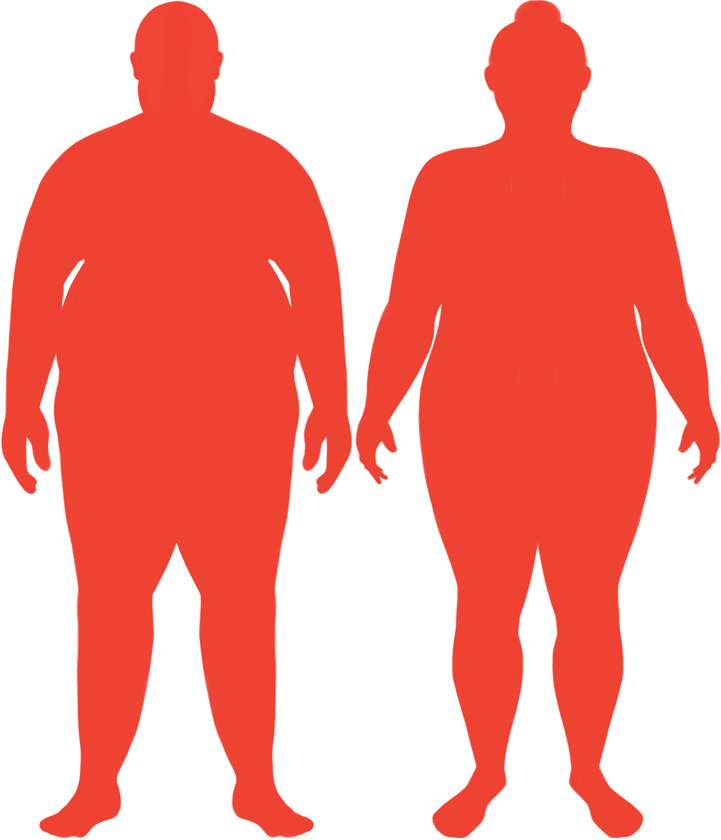# BMI Calculator

Result for 6'0" and 300 lbs.
Change Here.40.68

### Obese BMI

At 6'0" and 300 Pounds your BMI is 40.68

### BMI Scale

 Under Normal Over Obese
-79 lbs
to reach an
overweight bmi classification.
##### Overweight BMI Ends:
221.2 lbs
-116 lbs
to reach a
normal bmi classification.
184.3 lbs

### BMI Grading Table2 for Height 6'0"

 Weight Weight Class Below 136.4 lbs Underweight 136.4 lbs - 184.3 lbs Normal Weight 184.3 lbs - 221.2 lbs Overweight 221.2 lbs and Above Obese

### Weight Loss

If you were seeking a normal BMI weight of 184.3lbs, and you lost two pounds a week4, then you could reach a normal BMI in
58 Weeks
Or to be considered overweight, a goal weight of 221.2 lbs could be reached in
40 Weeks

### Obese BMI Silhouette### Ideal Weight for a 6 foot 0 Male or Female2

The ideal weight range using the BMI for a male or female with a height of 6'0" is

### Between

136.4 lbs

and
184.3 lbs
How to Calculate BMI3 for 6'0" and 300 lbs
(6 x 12) + 0 = 72
(300 / (72 x 72)) x 703
= 40.68287037037
6 = foot | 0 = inch | 300 = weight lbs

### Conversion

• 6 foot 0 = 72 inches
• 6 foot 0 = 182.88cm
• 300 pounds = 21 stone 6 pounds
• 300 pounds = 136.08 kg
• 300 pounds = 136077.71 grams

### 6'0" and 300 lbs Summary

What is the BMI for a 6'0" and 300 lbs female? 40.68 BMI, Obese.
What is the BMI for a 6'0" and 300 lbs male? 40.68 BMI, Obese.
What is the ideal weight for a 6'0" female? Between: 136.4lbs and 184.3lbs
What is the ideal weight for a 6'0" male? Between: 136.4lbs and 184.3lbs
If I am 6ft 0in and weigh 300 lbs, is that a good weight for my height? Under the BMI classification, 300 lbs is classed as being Obese.